# Train Neural ODE Network

This example shows how to train an augmented neural ordinary differential equation (ODE) network.

A neural ODE  is a deep learning operation that returns the solution of an ODE. In particular, given an input, a neural ODE operation outputs the numerical solution of the ODE $\mathit{y}\prime =\mathit{f}\left(\mathit{t},\mathit{y},\theta \text{\hspace{0.17em}}\right)$ for the time horizon $\left({t}_{0},{t}_{1}\right)$ and the initial condition $y\left({t}_{0}\right)={y}_{0}$, where $t$ and $y$ denote the ODE function inputs and $\theta$ is a set of learnable parameters. Typically, the initial condition ${y}_{0}$ is either the network input or, as in the case of this example, the output of another deep learning operation.

An augmented neural ODE  operation improves upon a standard neural ODE by augmenting the input data with extra channels and then discarding the augmentation after the neural ODE operation. Empirically, augmented neural ODEs are more stable, generalize better, and have a lower computational cost than neural ODEs.

This example trains a simple convolutional neural network with an augmented neural ODE operation.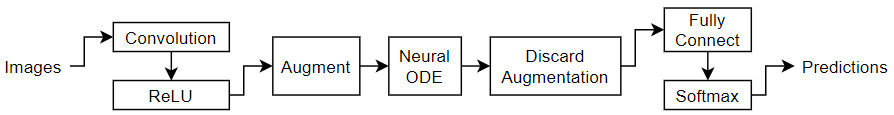The ODE function can be a collection of deep learning operations. In this example, the model uses a convolution-tanh block as the ODE function: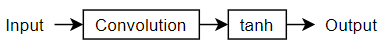The example shows how to train a neural network to classify images of digits using an augmented neural ODE operation.

Load the training images and labels using the `digitTrain4DArrayData` function.

`[XTrain,TTrain] = digitTrain4DArrayData;`

View the number of classes of the training data.

```classNames = categories(TTrain); numClasses = numel(classNames)```
```numClasses = 10 ```

View some images from the training data.

```numObservations = size(XTrain,4); idx = randperm(numObservations,64); I = imtile(XTrain(:,:,:,idx)); figure imshow(I)```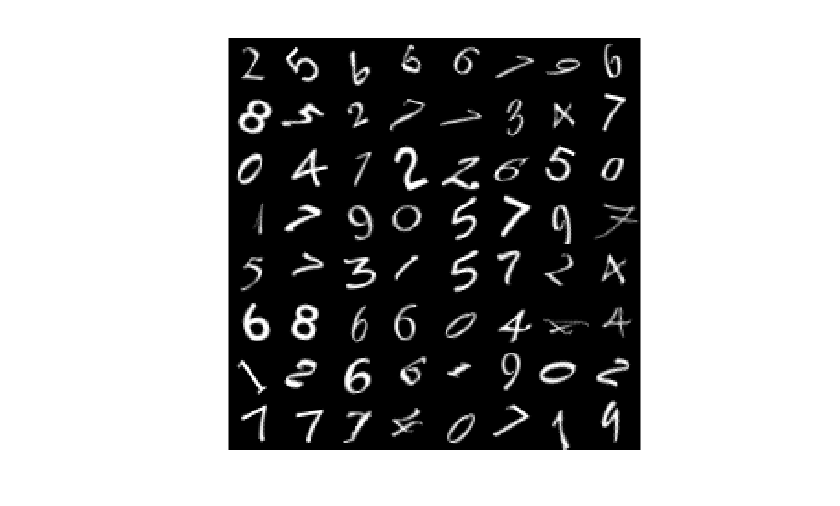### Define Deep Learning Model

Define the following network, which classifies images.

• A convolution-ReLU block with 8 3-by-3 filters with a stride of 2

• An augmentation step that concatenates an array of zeros to the input such that the number of channels is doubled

• A neural ODE operation with ODE function containing a convolution-tanh block with 16 3-by-3 filters

• For classification output, a fully connect operation of size 10 (the number of classes) and a softmax operationA neural ODE operation outputs the solution of a specified ODE function. For this example, specify a convolution-tanh block as the ODE function.That is, specify the ODE function given by $\mathit{y}\prime =\mathit{f}\left(\mathit{t},\mathit{y},\theta \text{\hspace{0.17em}}\right)$, where $f$ denotes the convolution-tanh operation, $y$ is the input data, and $\theta$ contains the learnable parameters for the convolution operation. In this case, the variable $t$ is unused.

#### Define and Initialize Model Parameters

Define the learnable parameters for each of the operations and include them in a structure. Use the format `parameters.OperationName.ParameterName`, where `parameters` is the structure, O`perationName` is the name of the operation (for example, "conv1"), and `ParameterName` is the name of the parameter (for example, "Weights"). Initialize the learnable layer weights and biases using the `initializeGlorot` and `initializeZeros` example functions, respectively. The initialization example functions are attached to this example as supporting files. To access these functions, open this example as a live script. For more information about initializing learnable parameters for model functions, see Initialize Learnable Parameters for Model Function.

Initialize the parameters structure.

`parameters = struct;`

Initialize the parameters for the first convolutional layer. Specify 8 3-by-3 filters. If you change these dimensions, then you must manually calculate the input size of the fully connect operation for its Glorot weights initialization.

```filterSize = [3 3]; numFilters = 8; numChannels = size(XTrain,3); sz = [filterSize numChannels numFilters]; numOut = prod(filterSize) * numFilters; numIn = prod(filterSize) * numFilters; parameters.conv1.Weights = initializeGlorot(sz,numOut,numIn); parameters.conv1.Bias = initializeZeros([numFilters 1]);```

Initialize the parameters for the convolution operation used in the neural ODE function. Because the augmentation step augments the input data with an array of zeros, the number of input channels is given by `numFilters + numExtraChannels`, where `numExtraChannels` is the number of channels in the augmentation. Similarly, because the model discards channels of the output of the neural ODE operation corresponding to the augmentation, the convolution operation in the neural ODE must have (`numChannels + numExtraChannels`) filters, where `numChannels` is the desired number of output channels.

Specify the same number of filters as the first convolution layer and a matching augmentation size.

```numChannels = numFilters; numExtraChannels = numFilters; numFiltersAugmented = numChannels + numExtraChannels; sz = [filterSize numFiltersAugmented numFiltersAugmented]; numOut = prod(filterSize) * numFiltersAugmented; numIn = prod(filterSize) * numFiltersAugmented; parameters.neuralode.Weights = initializeGlorot(sz,numOut,numIn); parameters.neuralode.Bias = initializeZeros([numFiltersAugmented 1]);```

Initialize the parameters for the fully connect operation. To initialize the weights of the fully connect operation using the Glorot initializer, first calculate the number of input elements to the operation.

For each operation in the model that changes the size of the data flowing through, consider the output sizes when you pass 28-by-28 images through the model:

• The first convolution has 8 filters with `"same"` padding and a stride of 2. This operation outputs 14-by-14 images with 8 channels.

• The model then augments the data with an 8-channel array of zeros. This operation outputs 14-by-14 images with 16 channels.

• The neural ODE operation has a convolution operation with 16 filters and `"same"` padding. This operation outputs 14-by-14 images with 16 channels.

• The model then discards the channels corresponding to the augmentation. This operation outputs 14-by-14 images with 8 channels.

This means that the number of input elements to the fully connect operation is $14*14*8=1568$.

```sz = [14 14]; inputSize = prod(sz)*numChannels; outputSize = numClasses; sz = [outputSize inputSize]; numOut = outputSize; numIn = inputSize; parameters.fc1.Weights = initializeGlorot(sz,numOut,numIn); parameters.fc1.Bias = initializeZeros([outputSize 1]);```

View the structure of parameters.

`parameters`
```parameters = struct with fields: conv1: [1×1 struct] neuralode: [1×1 struct] fc1: [1×1 struct] ```

View the parameters for the neural ODE operation.

`parameters.neuralode`
```ans = struct with fields: Weights: [3×3×16×16 dlarray] Bias: [16×1 dlarray] ```

#### Define Model Hyper Parameters

Define the hyperparameters for the operations and include them in a structure. Use the format `hyperparameters.OperationName.ParameterName` where `hyperparameters` is the structure, O`perationName` is the name of the operation (for example "neuralode") and `ParameterName` is the name of the hyperparameter (for example, "tspan").

Initialize the hyperparameters structure.

`hyperparameters = struct;`

For the neural ODE, specify an interval of integration of [0 0.1].

`hyperparameters.neuralode.tspan = [0 0.1];`

#### Define Neural ODE Function

Create the function `odeModel`, listed in the ODE Function section of the example, which takes as input the time input (unused), the initial conditions, and the ODE function parameters. The function applies a convolution operation followed by a tanh operation to the input data using the weights and biases given by the parameters.

#### Define Model Function

Create the function `model`, listed in the Model Function section of the example, which computes the outputs of the deep learning model. The function `model` takes as input the model parameters and the input data. The function outputs the predictions for the labels.

#### Define Model Loss Function

Create the function `modelLoss`, listed in the Model Loss Function section of the example, which takes as input the model parameters and a mini-batch of input data with corresponding targets containing the labels, and returns the loss and the gradients of the loss with respect to the learnable parameters.

### Specify Training Options

Specify the training options. Train with a mini-batch size of 64 for 30 epochs.

```miniBatchSize = 64; numEpochs = 30;```

### Train Model

Train the model using a custom training loop.

Create a `minibatchqueue` object that processes and manages mini-batches of images during training. To create a `minibatchqueue` object, first create a datastore that returns the images and labels by creating array datastores and then combining them.

```dsXTrain = arrayDatastore(XTrain,IterationDimension=4); dsTTrain = arrayDatastore(TTrain); dsTrain = combine(dsXTrain,dsTTrain);```

Create the mini-batch queue. For each mini-batch:

• Use the custom mini-batch preprocessing function `preprocessMiniBatch`, defined in the Mini-Batch Preprocessing Function section of the example, to convert the labels to one-hot encoded variables.

• Format the image data with the dimension labels "`SSCB"` (spatial, spatial, channel, batch). By default, the `minibatchqueue` object converts the data to `dlarray` objects with underlying type `single`.

• Train on a GPU if one is available. By default, the `minibatchqueue` object converts each output to a `gpuArray` if a GPU is available. Using a GPU requires Parallel Computing Toolbox™ and a supported GPU device. For information on supported devices, see GPU Support by Release (Parallel Computing Toolbox).

```mbq = minibatchqueue(dsTrain, ... MiniBatchSize=miniBatchSize, ... MiniBatchFcn=@preprocessMiniBatch, ... MiniBatchFormat=["SSCB" "CB"]);```

Initialize the moving average of the parameter gradients and the element-wise squares of the gradients used by the Adam optimizer.

```trailingAvg = []; trailingAvgSq = [];```

Initialize the training plot.

```figure C = colororder; lineLossTrain = animatedline(Color=C(2,:)); ylim([0 inf]) xlabel("Iteration") ylabel("Loss") grid on```

Train the model using a custom training loop. For each epoch, shuffle the data. For each mini-batch:

• Evaluate the model loss and gradients using the `dlfeval` and `modelLoss` functions.

• Update the network parameters using the `adamupdate` function.

• Update the training progress plot.

```iteration = 0; start = tic; % Loop over epochs. for epoch = 1:numEpochs % Shuffle data. shuffle(mbq) % Loop over mini-batches. while hasdata(mbq) iteration = iteration + 1; [X,T] = next(mbq); % Evaluate the model loss and gradients using dlfeval and the % modelLoss function. [loss,gradients] = dlfeval(@modelLoss, parameters, X, T, hyperparameters); % Update the network parameters using the Adam optimizer. [parameters,trailingAvg,trailingAvgSq] = adamupdate(parameters,gradients, ... trailingAvg,trailingAvgSq,iteration); % Display the training progress. D = duration(0,0,toc(start),Format="hh:mm:ss"); loss = double(gather(extractdata(loss))); addpoints(lineLossTrain,iteration,loss) title("Epoch: " + epoch + ", Elapsed: " + string(D)) drawnow end end```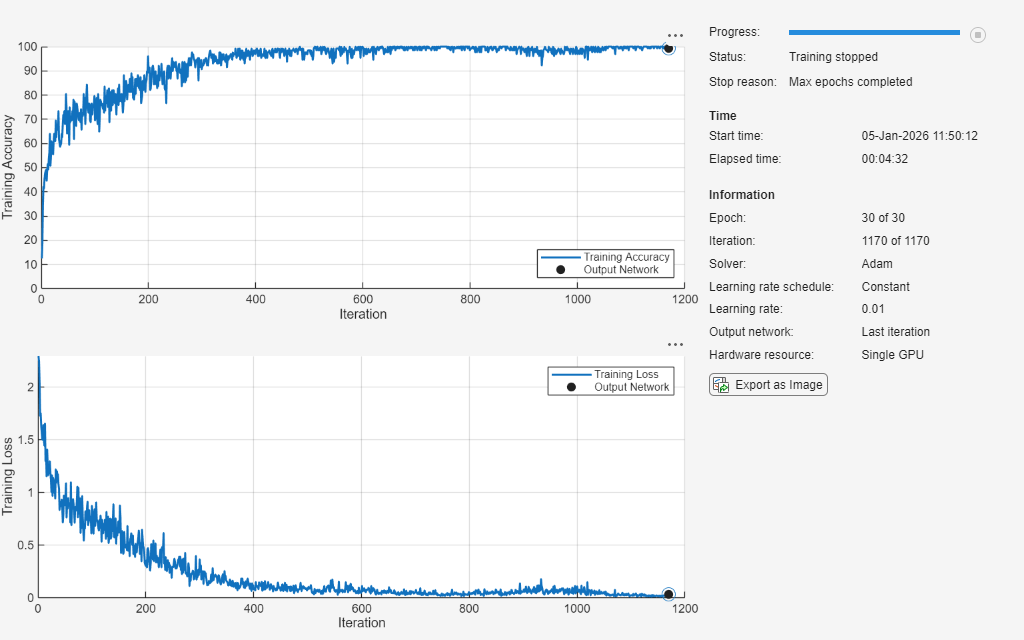### Test Model

Test the classification accuracy of the model by comparing the predictions on a held-out test set with the true labels.

`[XTest,TTest] = digitTest4DArrayData;`

After training, making predictions on new data does not require the labels. Create a `minibatchqueue` object containing only the predictors of the test data:

• Set the number of outputs of the mini-batch queue to 1.

• Specify the same mini-batch size used for training.

• Preprocess the predictors using the `preprocessPredictors` function, listed in the Mini-Batch Predictors Preprocessing Function section of the example.

• For the single output of the datastore, specify the mini-batch format "`SSCB"` (spatial, spatial, channel, batch).

```dsTest = arrayDatastore(XTest,IterationDimension=4); mbqTest = minibatchqueue(dsTest,1, ... MiniBatchSize=miniBatchSize, ... MiniBatchFormat="SSCB", ... MiniBatchFcn=@preprocessPredictors);```

Loop over the mini-batches and classify the sequences using `modelPredictions` function, listed in the Model Predictions Function section of the example.

`YPred = modelPredictions(parameters,hyperparameters,mbqTest,classNames);`

Visualize the predictions in a confusion matrix.

```figure confusionchart(TTest,YPred)```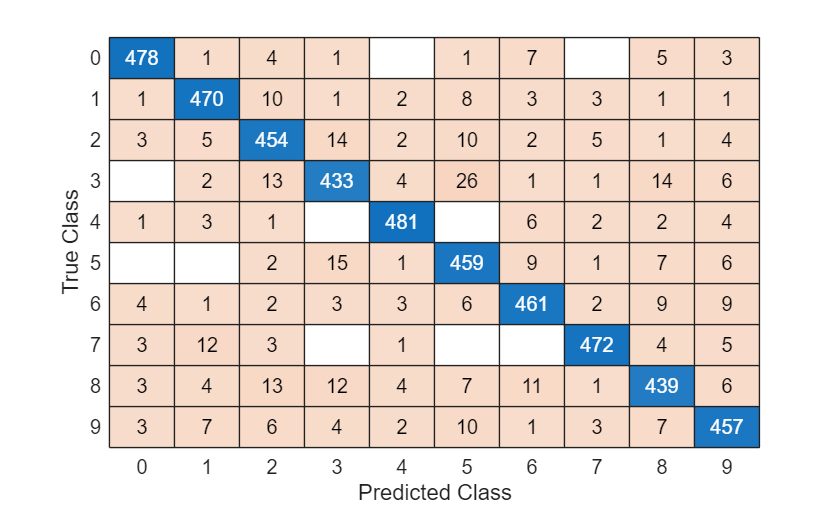### Model Function

The function `model` takes as input the model parameters, the input data `X`, the model hyperparameters, and outputs the predictions for the labels.

This diagram outlines the model structure.For the neural ODE operation, use the `dlode45` function and specify the `odeModel` function, listed in the ODE Function section of the example. Increase the absolute and relative tolerance using the `AbsoluteTolerance` and `RelativeTolerance` name-value arguments, respectively. To calculate the gradients by solving the associated adjoint ODE system, set the `GradientMode` option to `"adjoint"`.

```function Y = model(parameters,X,hyperparameters) % Convolution, ReLU. weights = parameters.conv1.Weights; bias = parameters.conv1.Bias; Y = dlconv(X,weights,bias,Padding="same",Stride=2); Y = relu(Y); % Augment. weights = parameters.neuralode.Weights; numChannels = size(Y,3); szAugmented = size(Y); szAugmented(3) = size(weights,3) - numChannels; Y0 = cat(3, Y, zeros(szAugmented,"like",Y)); % Neural ODE. tspan = hyperparameters.neuralode.tspan; Y = dlode45(@odeModel,tspan,Y0,parameters.neuralode, ... GradientMode="adjoint", ... AbsoluteTolerance=1e-3, ... RelativeTolerance=1e-4); % Discard augmentation. Y(:,:,numChannels+1:end,:) = []; % Fully connect, softmax. weights = parameters.fc1.Weights; bias = parameters.fc1.Bias; Y = fullyconnect(Y,weights,bias); Y = softmax(Y); end```

### ODE Function

The neural ODE operation consists of a convolution operation followed by a tanh operation.The ODE function `odeModel` takes as input the function inputs `t` (unused) and `y` and the ODE function parameters `p` containing the convolution weights and biases, and returns the output of the convolution-tanh block operation.

```function z = odeModel(t,y,p) weights = p.Weights; bias = p.Bias; z = dlconv(y,weights,bias,Padding="same"); z = tanh(z); end```

### Model Loss Function

The `modelLoss` function takes as input the model parameters, a mini-batch of input data `X` with corresponding targets `T`, and model hyperparameters, and returns the gradients of the loss with respect to the learnable parameters and the corresponding loss. To compute the gradients using automatic differentiation, use the `dlgradient` function.

```function [loss,gradients] = modelLoss(parameters,X,T,hyperparameters) Y = model(parameters,X,hyperparameters); loss = crossentropy(Y,T); gradients = dlgradient(loss,parameters); end```

### Model Predictions Function

The `modelPredictions` function takes as input the model parameters, model hyperparameters, a `minibatchqueue` of input data `mbq`, and the network classes, and computes the model predictions by iterating over all data in the `minibatchqueue` object. The function uses the `onehotdecode` function to find the predicted classes with the highest score.

```function predictions = modelPredictions(parameters,hyperparameters,mbq,classNames) predictions = []; while hasdata(mbq) X = next(mbq); Y = model(parameters,X,hyperparameters); Y = onehotdecode(Y,classNames,1)'; predictions = [predictions; Y]; end end```

### Mini-Batch Preprocessing Function

The `preprocessMiniBatch` function preprocesses a mini-batch of predictors and labels using the following steps:

1. Preprocess the images using the `preprocessPredictors` function.

2. Extract the label data from the incoming cell array and concatenate into a categorical array along the second dimension.

3. One-hot encode the categorical labels into numeric arrays. Encoding into the first dimension produces an encoded array that matches the shape of the network output.

```function [X,T] = preprocessMiniBatch(dataX,dataT) % Preprocess predictors. X = preprocessPredictors(dataX); % Extract label data from cell and concatenate. T = cat(2,dataT{:}); % One-hot encode labels. T = onehotencode(T,1); end```

### Predictors Preprocessing Function

The `preprocessPredictors` function preprocesses a mini-batch of predictors by extracting the image data from the input cell array and concatenating the data into a numeric array. For grayscale input, concatenating over the fourth dimension adds a third dimension to each image to use as a singleton channel dimension.

```function X = preprocessPredictors(dataX) X = cat(4,dataX{:}); end```

### Bibliography

1. Chen, Ricky T. Q., Yulia Rubanova, Jesse Bettencourt, and David Duvenaud. “Neural Ordinary Differential Equations.” Preprint, submitted June 19, 2018. https://arxiv.org/abs/1806.07366.

2. Dupont, Emilien, Arnaud Doucet, and Yee Whye Teh. “Augmented Neural ODEs.” Preprint, submitted October 26, 2019. https://arxiv.org/abs/1904.01681.